## ThinkDSP¶

This notebook contains code examples from Chapter 4: Noise

In :
# Get thinkdsp.py

import os

if not os.path.exists('thinkdsp.py'):
!wget https://github.com/AllenDowney/ThinkDSP/raw/master/code/thinkdsp.py

In :
import numpy as np
import matplotlib.pyplot as plt

from thinkdsp import decorate

In :
np.random.seed(17)


The simplest noise to generate is uncorrelated uniform (UU) noise:

In :
from thinkdsp import UncorrelatedUniformNoise

signal = UncorrelatedUniformNoise()
wave = signal.make_wave(duration=0.5, framerate=11025)
wave.make_audio()

Out:

Here's what a segment of it looks like:

In :
segment = wave.segment(duration=0.1)
segment.plot()
decorate(xlabel='Time (s)',
ylabel='Amplitude')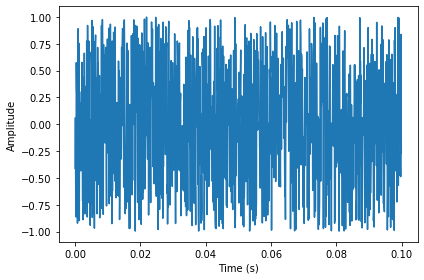In [ ]:



And here's the spectrum:

In :
spectrum = wave.make_spectrum()
spectrum.plot(linewidth=0.5)
decorate(xlabel='Frequency (Hz)',
ylabel='Amplitude')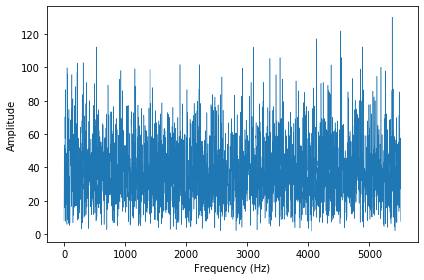In the context of noise it is more conventional to look at the spectrum of power, which is the square of amplitude:

In :
spectrum.plot_power(linewidth=0.5)
decorate(xlabel='Frequency (Hz)',
ylabel='Power')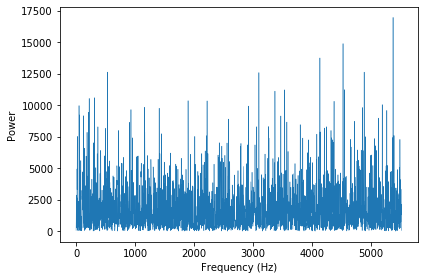UU noise has the same power at all frequencies, on average, which we can confirm by looking at the normalized cumulative sum of power, which I call an integrated spectrum:

In :
integ = spectrum.make_integrated_spectrum()
integ.plot_power()
decorate(xlabel='Frequency (Hz)',
ylabel='Cumulative power')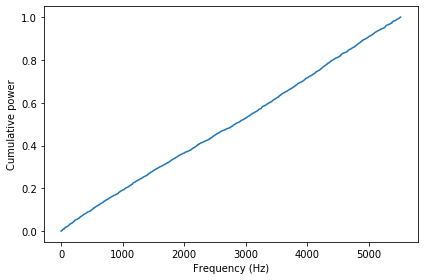A straight line in this figure indicates that UU noise has equal power at all frequencies, on average. By analogy with light, noise with this property is called "white noise".

### Brownian noise¶

Brownian noise is generated by adding up a sequence of random steps.

In :
from thinkdsp import BrownianNoise

signal = BrownianNoise()
wave = signal.make_wave(duration=0.5, framerate=11025)
wave.make_audio()

Out:

The sound is less bright, or more muffled, than white noise.

Here's what the wave looks like:

In :
wave.plot(linewidth=1)
decorate(xlabel='Time (s)',
ylabel='Amplitude')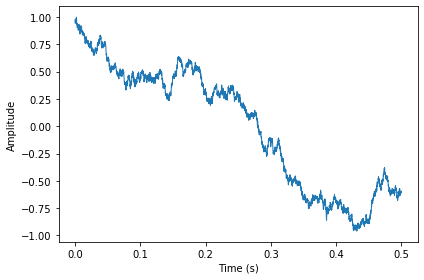Here's what the power spectrum looks like on a linear scale.

In :
spectrum = wave.make_spectrum()
spectrum.plot_power(linewidth=0.5)
decorate(xlabel='Frequency (Hz)',
ylabel='Power')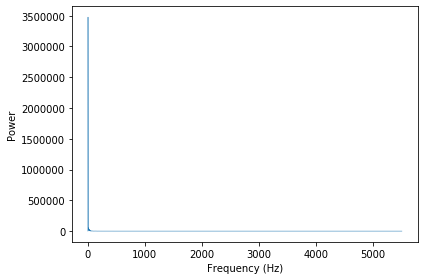So much of the energy is at low frequencies, we can't even see the high frequencies.

We can get a better view by plotting the power spectrum on a log-log scale.

In :
# The f=0 component is very small, so on a log scale
# it's very negative.  If we clobber it before plotting,
# we can see the rest of the spectrum more clearly.
spectrum.hs = 0

spectrum.plot_power(linewidth=0.5)

loglog = dict(xscale='log', yscale='log')
decorate(xlabel='Frequency (Hz)',
ylabel='Power',
**loglog)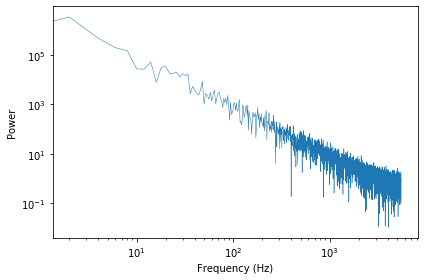Now the relationship between power and frequency is clearer. The slope of this line is approximately -2, which indicates that $P = K / f^2$, for some constant $K$.

In :
signal =  BrownianNoise()
wave = signal.make_wave(duration=0.5, framerate=11025)
spectrum = wave.make_spectrum()
result = spectrum.estimate_slope()
result.slope

Out:
-1.7846032211221763

The estimated slope of the line is closer to -1.8 than -2, for reasons we'll see later.

### Pink noise¶

Pink noise is characterized by a parameter, $\beta$, usually between 0 and 2. You can hear the differences below.

With $\beta=0$, we get white noise:

In :
from thinkdsp import PinkNoise

signal = PinkNoise(beta=0)
wave = signal.make_wave(duration=0.5)
wave.make_audio()

Out:

With $\beta=1$, pink noise has the relationship $P = K / f$, which is why it is also called $1/f$ noise.

In :
signal = PinkNoise(beta=1)
wave = signal.make_wave(duration=0.5)
wave.make_audio()

Out:

With $\beta=2$, we get Brownian (aka red) noise.

In :
signal = PinkNoise(beta=2)
wave = signal.make_wave(duration=0.5)
wave.make_audio()

Out:

The following figure shows the power spectrums for white, pink, and red noise on a log-log scale.

In :
betas = [0, 1, 2]

for beta in betas:
signal = PinkNoise(beta=beta)
wave = signal.make_wave(duration=0.5, framerate=1024)
spectrum = wave.make_spectrum()
spectrum.hs = 0
label = f'beta={beta}'
spectrum.plot_power(linewidth=1, alpha=0.7, label=label)

decorate(xlabel='Frequency (Hz)',
ylabel='Power',
**loglog)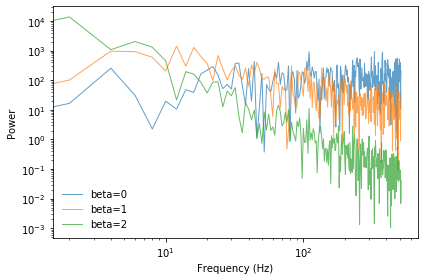### Uncorrelated Gaussian noise¶

An alternative to UU noise is uncorrelated Gaussian (UG noise).

In :
from thinkdsp import UncorrelatedGaussianNoise

signal = UncorrelatedGaussianNoise()
wave = signal.make_wave(duration=0.5, framerate=11025)
wave.plot(linewidth=0.5)
decorate(xlabel='Time (s)',
ylabel='Amplitude')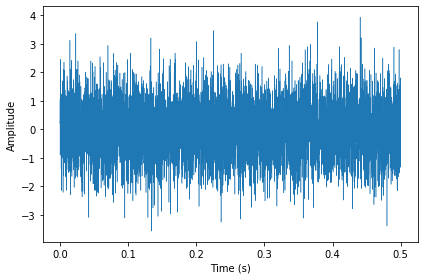The spectrum of UG noise is also UG noise.

In :
spectrum = wave.make_spectrum()
spectrum.plot_power(linewidth=1)
decorate(xlabel='Frequency (Hz)',
ylabel='Power')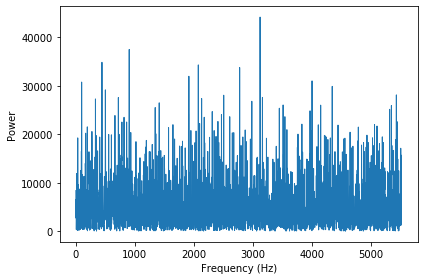We can use a normal probability plot to test the distribution of the power spectrum.

In :
def normal_prob_plot(sample, fit_color='0.8', **options):
"""Makes a normal probability plot with a fitted line.

sample: sequence of numbers
fit_color: color string for the fitted line
options: passed along to Plot
"""
n = len(sample)
xs = np.random.normal(0, 1, n)
xs.sort()

ys = np.sort(sample)

mean, std = np.mean(sample), np.std(sample)
fit_ys = mean + std * xs
plt.plot(xs, fit_ys, color='gray', alpha=0.5, label='model')

plt.plot(xs, ys, **options)

In :
normal_prob_plot(spectrum.real, color='C0', label='real part')
decorate(xlabel='Normal sample',
ylabel='Power')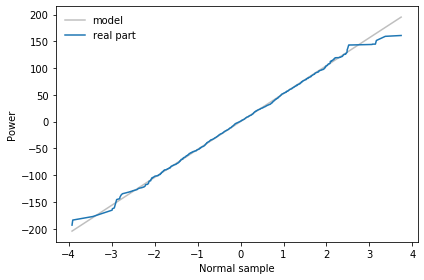A straight line on a normal probability plot indicates that the distribution of the real part of the spectrum is Gaussian.

In :
normal_prob_plot(spectrum.imag, color='C1', label='imag part')
decorate(xlabel='Normal sample',
ylabel='Power')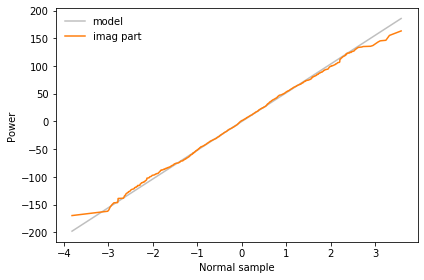And so is the imaginary part.

In [ ]: